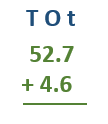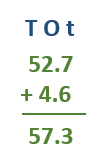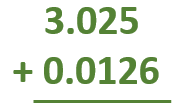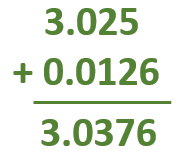Smartick is an advanced online program that teaches kids math and coding in only 15 min. a day

Dec14

# How to Add Decimal Numbers and Some Examples

In this post, we are going to learn how to add decimal numbers.

For example:

## 52.7 + 4.6

The most important thing about adding decimals is to put the decimal numbers in the correct position. For this, we have to align the decimals and be sure all digits of the same place value are in the same columns: tens place (T), ones place (O) and tenths place (t) in our example.Once positioned, we have only to add the two numbers: add the numbers in the same way as you add the numbers without the decimal. After you add the numbers, just bring the decimal straight down. So, in the end, the decimal is in the same location in the sum as it was in the addends:We are going to see another example:

## 3.025 + 0.0126

First, we have to align the numbers in the correct position: units in the same column and periods one below the other:Now, we add the numbers as if there were not a period and to finish the operation, we put the decimal in the same position that it was in the addends.Now, it’s up to you to practice!

You can register at Smartick, try it for free and learn much more elementary math.